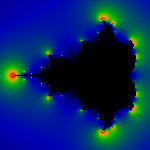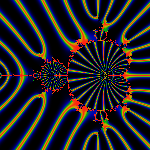The generalization of the integer iteration count gives us a handy tool for computing other quantities, including Douady-Hubbard rays. This is because the renormalized count can be used to provide a simple expression for the Douady-Hubbard potential. We give the the expression, and then prove that the potential is indeed harmonic, immediately below.

zn+1 = zn2+c

The renormalized iteration count is

m(R) = n+1 - log(log |zn|) / log 2

where we have picked an escape radius R, and defined n to be the smallest n such that R < |zn| . Then, as shown on the Escape Page, m(R) is 'almost' independent of R and n, in the sense that m approaches a well-defined limit as R->oo, and, in fact, converges very rapidly. It is also 'almost' continuous and smooth, in the sense that there are only a measure-zero of discontinuities for finite R, and that all discontinuities are bounded, and get vanishingly small in the limit of R->oo. That is, in the limit, m becomes continuous and smooth, and, as a practical, computational matter, is plenty smooth enough for small R (100 to 10000). Below, when we write m, we sometimes mean the limit m(R->oo) and sometimes m(R) for finite R, and most of the statements apply to either.

f = e-m log2 = 2-m

Let us now show that f is harmonic, both in the limit R->oo and for finite R, and that it has the right asymptotic behavior. The limitations of html make this presentation hard. Please bear with us as we define some basic notation.

Lets define the derivative w.r.t a complex variable c=x+iy in the usual fashion:

D = d/dc = (d/dx - id/dy)/2

and

D = d/dc = (d/dx + id/dy)/2

where we use an underline to denote complex conjugate (because 'overline', the usual way of showing this, is not available in html). The harmonic or Laplacian is then just

4 DD = d2/dx2 + d2/dy2

Lets review some trivial factoids to make sure we're on the same page. Since zn(c,c) = zn(c) is not a function of c, we have Dz = Dz = 0 . The modulus is just |z| = sqrt(zz), and the derivative of the modulus is D|z| = z Dz / (2|z|) . We can now combine these to compute the Laplacian of the the renormalized iteration count:

4 DD m = Dzn Dzn / (log 2 |zn|2 log2 |zn|)

Note that this depends implicitly on the escape radius R, since by definition, R < |zn|. Thus, it would seem that the harmonic can be made arbitrarily small, provided the numerator was bounded. Unfortunately, it is not; not only is it unbounded, but it diverges 'worse than exponentially' as one approaches the boundary of the M-set.

The Laplacian of the potential is

4 DD f = 4f (log22 Dm Dm - log2 DDm)

But

Dm = D|zn| / (log2 |zn| log |zn|)

Substituting from the above, we can easily see

DD f = 0

both for finite R and R->oo. For finite R, f has 'small' discontinuities, which vanish in the limit; thus the harmonic is non-zero on the discontinuities, but these vanish in the limit, and f is harmonic everywhere outside the M-set. To complete this analysis of this function, we check the asymptotic behavior. For finite R, we can write f(R) = 2-(n+1) log |zn| . Thus, for c approaching the boundary of the M-set, n gets infinitely large, whereas |zn| < R2 and so f=0 on the boundary. At the other end, for large c, we can approximate zn+1 ~zn2 for any n, and so

m ~ 2 - log(log |c|) / log 2

and

f ~ log |c| / 4

for large c.

Now that we have f, we should play a few games. We could draw a picture of f, but that in fact is 'boring'. f gets exponentially flat as it approaches the boundary of the M-set, and thus, it looks like a very blurry low-res picture of the M-set. Boring.

2Df = f zn Dzn / |zn|2 log |zn|

The picture below shows the modulus of the gradient.Of course, it resembles a coronal discharge. If one were to cut out a metal plate in the shape of an M-set, apply a high voltage to it, it is exactly this that you would see, because it is the areas of high electric field (gradient) where the coronal ionization will occur. Maybe make a chic lightning rod for the barn.

The next picture encodes the angle of the landing rays on the M-set.It is nothing more than the phase-angle of the gradient. The color coding is such that black=0, blue=small angle, green=pi, red=2pi. In other words, green points west, and red/black points east, just as it was in the Soviet era.

This last image shows some landing rays.A 'landing ray' is just the integral flow along the gradient lines of the potential; i.e. the path a rock rolling down the hill to the M-set would take. We've picked 31 evenly-spaced angles at infinity; the red lines indicate the flow along these paths. The color gradations help show the behavior of some neighboring rays: i.e. the blue rays are nearby neighbors of the red rays. The spreading as they 'land' on the M-set just helps show that rays become radically divergent. The Mandelbrot interior is an artifact of the algorithm, and are not a part of the Douady-Hubbard isomorphism. See the Winding Page for more details on the derivation, and more pictures.

References

• John Milnor, Periodic Orbits, External Rays and the Mandelbrot Set: An Expository Account, (postscript, mirror of StonyBrook preprint).

Created by Linas Vepstas December 2000
linas@linas.org